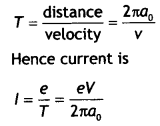Here we are providing Class 12 Physics Important Extra Questions and Answers Chapter 12 Atoms. Important Questions for Class 12 Physics with Answers are the best resource for students which helps in Class 12 board exams.

## Class 12 Physics Chapter 12 Important Extra Questions Atoms

### Atoms Important Extra Questions Very Short Answer Type

Question 1.
Name the spectral series which lies in the visible region.
Balmer series.

Question 2.
What is the maximum number of spectral lines emitted by a hydrogen atom when it is in the third excited state? (CBSE AI 2013C)
Six.

Question 3.
When is Hα line of the Balmer series in the emission spectrum of hydrogen atom obtained? (CBSE Delhi 2013C)
It is obtained when an electron jumps from n =3 to n = 2 level.

Question 4.
A mass of lead is embedded in a block of wood. Radiations from a radioactive source incident on the side of the block produce a shadow on a fluorescent screen placed beyond the block. The shadow of the wood is faint but the shadow of lead is dark. Give a reason for this difference.

Question 5.
What was the source of alpha particles in Rutherford’s alpha scattering experiment?
The source was 21483Bi.

Question 6.
If the radius of the ground level of a hydrogen atom is 5.3 nm, what is the radius of the first excited state?
It is 4 × 5.3 = 21.2 nm ( ∵ r = n²ro)

Question 7.
Calculate the ratio of energies of photons produced due to the transition of electron of a hydrogen atom from its
(a) Second permitted energy level to the first level, and
energy of photon E1 = – 3.4 – (-13.6) = 10.2 eV

(b) Highest permitted energy level to the second permitted level.
energy of photon E2 = 0 – (-3.4) = 3.4 eV
Ratio $$\frac{E_{1}}{E_{2}}=\frac{10.2}{3.4}$$ = 3

Question 8.
The mass of an H-atom is less than the sum of the masses of a proton and electron. Why is this? (NCERT Exemplar) Answer:
Einstein’s mass-energy equivalence gives E = mc². Thus the mass of an H-atom is mp + me – B/c² where B ≈ 13.6 eV

### Atoms Important Extra Questions Short Answer Type

Question 1.
Define electron-volt and atomic mass unit. Calculate the energy in joule equivalent to the mass of one proton.
Electron volt: It is defined as the energy gained by an electron when accelerated through a potential difference of 1 volt. Atomic mass unit: It is defined as one-twelfth of the mass of one atom of carbon 12.

The mass of a proton is 1.67 × 10-27 kg. Therefore, energy equivalent of this mass is E = mc² = 1.67 × 10-27 × (3 × 108)2 = 1.5 × 10-10 J

Question 2.
State Bohr’s quantization condition of angular momentum. Calculate the shortest wavelength of the Bracket series and state to which part of the electromagnetic spectrum does it belong. (CBSE Delhi 2019)
Or
Calculate the orbital period of the electron in the first excited state of the hydrogen atom.
Bohr’s Quantisation condition: Only those orbits are permitted in which the angular momentum of the electron is an integral multiple of h/2π.

For Brackett Series,
The shortest wavelength is for the transition of electrons from ni = ∞ to nf = 4

Using the equation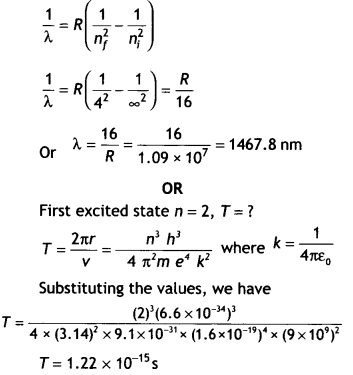Question 3.
Write two important limitations of the Rutherford nuclear model of the atom. (CBSE AI2018, Delhi 2018)

1. Rutherford’s model fails to explain the line spectra of the atom.
2. Rutherford’s model cannot explain the stability of the nucleus.

Question 4.
Find out the wavelength of the electron orbiting in the ground state of the hydrogen atom. (CBSEAI 2018, Delhi 2018)
The wavelength of an electron in the ground state of hydrogen atom is given by
E = $$\frac{hc}{λ}$$
or
λ = $$\frac{hc}{E}$$

For ground state
E = – 13.6 eV = 13.6 × 1.6 × 10-19 J

Hence wavelength of electron in the first orbit
λ = $$\frac{h c}{E}=\frac{6.6 \times 10^{-34} \times 3 \times 10^{8}}{13.6 \times 1.6 \times 10^{-19}}$$ = 0.9 × 10-7 J

Question 5.
(a) State Bohr’s postulate to define stable orbits in a hydrogen atom. How does de Broglie’s hypothesis explain the stability of these orbits?
Bohr’s postulate for stable orbits states the electron in an atom revolves around the nucleus only in those orbits for which its angular momentum is an integral multiple of h/2π (h = Planck’s constant), (n = 1, 2, 3 …)

As per de Broglie’s hypothesis λ = h/p = h/mv
For a stable orbit, we must have a circumference of the orbit = nλ (n = 1, 2, 3,…)
∴ 2πr = nλ
or
mvr = nh/2π

Thus de-Broglie showed that the formation . of stationary patterns for integral “n” gives rise to the stability of the atom.
This is nothing but Bohr’s postulate.

(b) A hydrogen atom initially in the ground state absorbs a photon which excites it to the n = 4 level. Estimate the frequency of the photon. (CBSE AI 2018, Delhi 2018)
Energy in the n = 4 level n1 = 1 and n2 = 4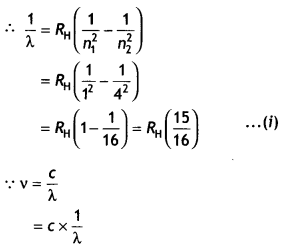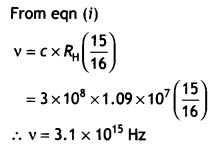Question 6.
An alpha particle moving with initial kinetic energy K towards a nucleus of atomic number Z approaches a distance ‘d’ at which it reverses its direction. Obtain an expression for the distance of closest approach ‘d’ in terms of the kinetic energy of the alpha particle, K. (CBSEAI2016C)
At the distance of the closest approach, the kinetic energy of the alpha particle is converted into the electrostatic potential energy of the alpha particle-nucleus system. Therefore, at the distance of the closest approach

we have
Kinetic energy = Potential energy
Therefore,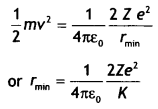where K is the kinetic energy.

Question 7.
The figure shows the energy level diagram of the hydrogen atom.(a) Find out the transition which results in the emission of a photon of wavelength 496 nm.
The wavelength of photon emitted is given by $$\frac{1}{λ}$$ = RH$$\left(\frac{1}{n_{\mathrm{f}}^{2}}-\frac{1}{n_{\mathrm{i}}^{2}}\right)$$

None of these transitions correspond to a wavelength of 496 nm. The closest is 4 to 2 of 489 nm

(b) Which transition corresponds to the emission of radiation of maximum wavelength? Justify your answer. (CBSE AI 2015 C)
Transition 4 to 3 as the frequency of this radiation is maximum.

Question 8.
A nucleus makes a transition from one permitted energy level to another level of lower energy. Name the region of the electromagnetic spectrum to which the emitted photon belongs. What is the order of its energy in electron-volts? Write four characteristics of nuclear forces.
(a) Emitted photon belongs to gamma-rays part of the electromagnetic spectrum.
(b) the energy is of the order of MeV.
(c) Four characteristics of nuclear forces are:

1. Nuclear forces are independent of charges.
2. Nuclear forces are short-range forces.
3. Nuclear forces are the strongest forces in nature, in their own small range of few fermis.
4. Nuclear forces are saturated forces.

### Atoms Important Extra Questions Long Answer Type

Question 1.
Explain Rutherford’s experiment on the scattering of alpha particles and state the significance of the results.
The schematic arrangement in the Geiger Marsden experiment is shown in the figure.Alpha-particles emitted by a Bismuth (21483Bi) radioactive source were collimated into a narrow beam by their passage through lead bricks. The beam was allowed to fall on a thin foil of gold of thickness 2.1 × 10-7 m. The scattered alpha-particles were observed through a rotatable detector consisting of a zinc sulfide screen and a microscope. The scattered alpha-particles on striking the screen produced bright light flashes or scintillations. These scintillations could be viewed through the microscope and counted at different angles from the direction of the incident beam.

Significance: The experiment established the existence of a nucleus that contained the entire positive charge and about 99.95% of the mass.

Question 2.
Using Bohr’s postulates, obtain the expression for the total energy of the electron in the stationary states of the hydrogen atom. Hence draw the energy level diagram showing how the line spectra corresponding to the Balmer series occur due to the transition between energy levels. (CBSE Delhi 2013)
The electron revolving around the nucleus has two types of energy:

Kinetic energy due to its motion.
Potential energy due to it lying in the electric field of the nucleus.

Thus the total energy of the electron is given by
E = K. E. + P. E. …(1)

An electron of mass m moving around the nucleus with an orbital velocity v has kinetic energy given by
K.E. = $$\frac{1}{2}$$mv² = $$\frac{1}{2} \frac{k e^{2}}{r}$$ …(2)

Now the potential energy of the electron at a distance r from the nucleus is given by
PE = potential due to the nucleus at a distance r × charge on the electron = V × – e …(3)

Now the potential at a distance r from the nucleus having a charge e is given by
V = k $$\frac{e}{r}$$ …..(4)

Substituting in equation (3) we have
P.E. = V × – e = -k$$\frac{e^{2}}{r}$$ …(5)

Substituting equations (2) and (3) in equation 1 we have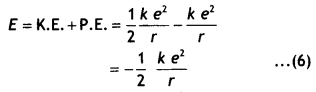But the radius of the nth orbit is given by
rn = $$\frac{n^{2} h^{2}}{4 \pi^{2} m e^{2} k}$$

Substituting in equation (6) we have
E = – $$\frac{2 \pi^{2} m e^{4} \mathrm{k}^{2}}{n^{2} \mathrm{~h}^{2}}$$ …(7)

This gives the expression for the energy possessed by the electron in the nth orbit of the hydrogen atom.

Question 3.
Hydrogen atoms are excited with an electron beam of energy of 12.5 eV. Find
(a) The highest energy level up to which the hydrogen atoms will be excited.
The maximum energy that the excited hydrogen atom can have is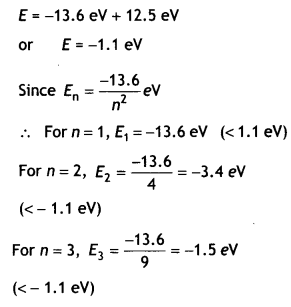For n=4, E4 = $$\frac{-13.6}{16}$$ = – 0.85eV
(> – 1.1 eV)

∴ The eLectron can only be excited up to n = 3 states.

(b) The longest wavelengths in the (i) Lyman series, (ii) Balmer series of the spectrum of these hydrogen atoms. (CBSE 2019C)
From energy tevet of hydrogen atom,
we have
$$\frac{1}{λ}$$ = R$$\left[\frac{1}{n_{1}^{2}}-\frac{1}{n_{2}^{2}}\right]$$

Longest wavelength of Lyman senesQuestion 4.
Using Bohr’s postulates of the atomic model derive the expression for the radius of the 11th electron orbit. Hence obtain the expression for Bohr’s radius. (CBSE AI 2014)
Let us consider a mechanical model of the hydrogen atom as shown in the figure that incorporates this quantization assumption.This atom consists of a single electron with mass m and charge – e revolving around a single proton of charge + e. The proton is nearly 2000 times as massive as the electron, so we can assume that the proton does not move. As the electron revolves around the nucleus the electrostatic force of attraction between the electron and the proton provides the necessary centripetal force. Therefore, we have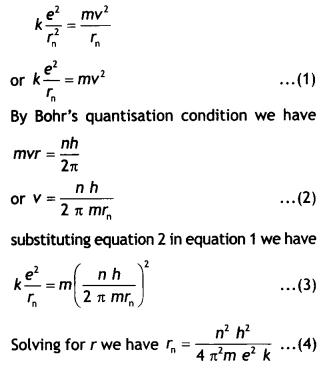This gives the radius of the nth orbit of the hydrogen atom.
If n = 1 we have r = ao which is called Bohr’s radius.
ao = $$\frac{h^{2}}{4 \pi^{2} m e^{2} k}$$

Question 5.
State Bohr’s postulate of the hydrogen atom successfully explains the emission lines in the spectrum of the hydrogen atoms.
Use the Rydberg formula to determine the wavelength of Ha line. [Given Rydberg constant R = 1.03 × 107 m-1] (CBSE AI 2015)
It states that an electron might make a transition from one of its specified non¬radiating orbits to another of lower energy. When it does so, a photon is emitted having energy equal to the energy difference between the initial and final states. The frequency of the emitted photon is then given by

hv = Ei – Ef where Ei and Ef are the energies of the initial and final states
Using the formula $$\frac{1}{λ}$$ = RH$$\left(\frac{1}{n_{f}^{2}}-\frac{1}{n_{i}^{2}}\right)$$ we have for Ha Line ni = 3 and nf = 2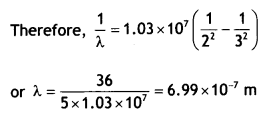Question 6.
Using Bohr’s postulates derive the expression for the frequency of radiation emitted when an electron In a hydrogen atom undergoes a transition from a higher energy state (quantum number n-) to the towering state (n,). When an electron in a hydrogen atom jumps from the energy state ni =4 to n = 3, 2, 1, identify the spectral series to which the emission lines belong. (CBSE Delhi 201 1C)
According to Bohr’s frequency condition, if an electron jumps from an energy Level E to E1, then the frequency of the emitted radiation is given by
hv = E – E1 …(1)

Let ni and nf be the corresponding orbits then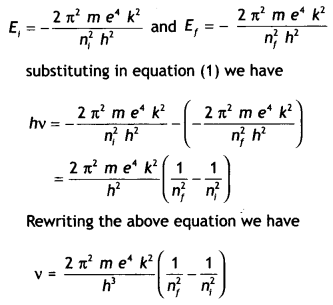This gives the frequency of the emitted radiation.
When ni =4 and nf = 3, Paschen series
When ni = 4 and nf = 2, Balmer series
When ni = 4 and nf = 1, Lyman senes

Question 7.
Calculate the ratio of the frequencies of the radiation emitted due to the transition of the electron In a hydrogen atom from Its (i) second permitted energy level to the first level and (ii) highest permitted energy level to the second permitted level. (CBSE Delhi 2018C)
We have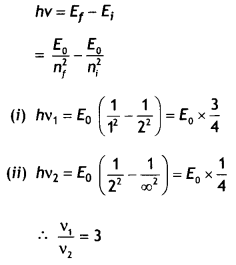Question 8.
Monochromatic radiation of wavelength 975 A excites the hydrogen atom from its ground state to a higher state. How many different spectral lines are possible In the resulting spectrum? Which transition corresponds to the longest wavelength amongst them? (CBSE Sample Paper 201819)
The energy corresponding to the given wavelength: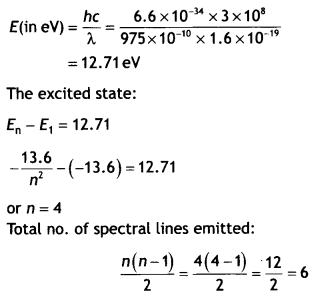The longest wavelength Will correspond to the transition n = 4 to n = 3

Question 9.
(a) Using postulates of Bohr’s theory of hydrogen atom, show that
(i) the radii of orbits increases as n², and
Let us consider a mechanical. model of the hydrogen atom as shown in the figure.This atom consists of a single electron with mass m and charge – e revolving around a single proton of charge + e. As the electron revolves around the nucleus the electrostatic force of attraction between the electron and the proton provides the necessary centripetal force. Therefore we have,Substituting equation 3 in equation 2 we haveThis gives the radius of the nth orbit of the hydrogen atom which shows that E ∝ $$\frac{1}{n^{2}}$$

(ii) the total energy of the electron increases as 1/n², where n is the principal quantum number of the atom.
the total energy possessed by an electron in the nth orbit of the hydrogen atom is given by
E = T + U …(1)
i.e. the sum of its kinetic and electrostatic potential energies.

An electron of mass m moving around the nucleus with an orbital velocity v has kinetic energy given by
K.E. = $$\frac{1}{2}$$mv² = $$\frac{1}{2}\frac{k e^{2}}{r}$$ ….(2)

Now the potential energy of the electron at a distance r from the nucleus is given by
PE = potential due to the nucleus at a distance r × charge on the electron
= V × – e …(3)

Now the potential at a distance r from the nucleus having a charge e is given by
V = k$$\frac{e}{r}$$ ….(4)

Substituting in equation 2 we have
P.E. = V × – e = – k $$\frac{e^{2}}{r}$$ …(5)

Substituting equations 2 and 5 in equation 1 we haveBut the radius of the nth orbit is given by rn = $$\frac{n^{2} h^{2}}{4 \pi^{2} m e^{2} k}$$

Substituting in equation 6 we have
E = – $$\frac{2 \pi^{2} m e^{4} k^{2}}{n^{2} h^{2}}$$ …(7)

This gives the expression for the energy possessed by the eLectron in the nth orbit of the hydrogen atom which shows that E ∝ $$\frac{1}{n^{2}}$$

(b) Calculate the wavelength of H2 line In Balmer series of hydrogen atom, given Rydberg constant R = 1.097 × 107 m-1. (CBSE AI 2011C)
For H2 Line in Balmer series n1 = 2 and n2 = 3Question 10.
State Bohr’s quantization condition for defining stationary orbits. How does de Brogue hypothesis explain the stationary orbits?
Find the relation between the three wavelengths λ1 λ2 and λ3 from the energy level diagram shown below. (CBSE Delhi 2016)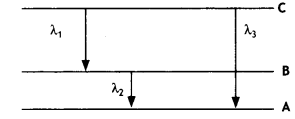It states that only those orbits are permitted in which the angular momentum of the electron about the nucleus is an integral multiple of $$\frac{h}{2π}$$, where his Planck’s constant.

According to de Broglie, an electron of mass m moving with speed v would have a wavelength λ given by
λ = h/mv.

Now according to Bohr’s postulate,
mvrn = $$\frac{n h}{2 \pi}$$
or
2πrn = $$\frac{nh}{mv}$$

But h / mv = A is the de BrogUe wavelength of the electron, therefore, the above equation becomes 2πrn = nλ where 2πrn is the circumference of the permitted orbit. If the wavelength of a wave does not close upon itself, destructive interference takes place as the wave travels around the loop and quickly dies out. Thus only waves that persist are those for which the circumference of the circular orbit contains a whole number of wavelengths.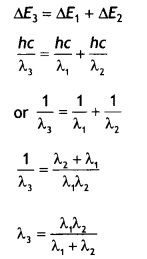Numerical Problem :
Formulae for solving numerical problems

• Distance of closest approach ro = $$\frac{1}{4 \pi \varepsilon_{0}} \frac{2 z E^{2}}{E_{k}}$$
• Radius of the nth orbit of hydrogen atom rn = $$\frac{n^{2} h^{2}}{4 \pi^{2} m e^{2} k}$$
• Velocity of eLectron in the ntt orbit v = $$v=\frac{c}{137 n}$$
• Wavelength of radiation emitted when electron jumps form ni to nf $$\frac{1}{\lambda}=R_{\mathrm{H}}\left(\frac{1}{n_{\mathrm{f}}^{2}}-\frac{1}{n_{\mathrm{i}}^{2}}\right)$$
• Energy of electron in the nth orbit of hydrogen atom
E = – $$\frac{2 \pi^{2} m e^{4} k^{2}}{n^{2} h^{2}}$$
or
E = – $$\frac{13.6}{n^{2}}$$ eV

Question 1.
A hydrogen atom in its excited state emits radiations of wavelengths 1218 Å and 974.3 Å when it finally comes to the ground state. Identify the energy levels from where transitions occur. Given Rydberg constant R = 1.1 × 107 m-1. Also, specify the spectral series to which these lines belong. (CBSE AI 2019)
We know that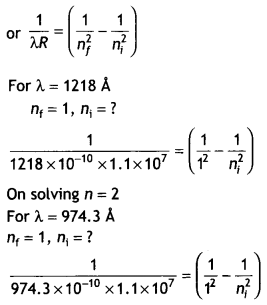On solving n = 4
Lyman series.

Question 2.
Obtain the first Bohr’s radius and the ground state energy of a muonic hydrogen atom, i.e. an atom where the electron is replaced by a negatively charged muon (μ) of mass about 207 me that orbits around a proton. (Given for hydrogen atom, the radius of first orbit and ground state energy are 0.53 × 10-10m and – 13.6 eV respectively) (CBSE AI 2019)
In Bohr’s model of hydrogen atom the radius of nth orbit is given by
rn = $$\frac{n^{2} h^{2}}{4 \pi^{2} e^{2} m_{e} k}$$

As n = 1
Therefore, we have 1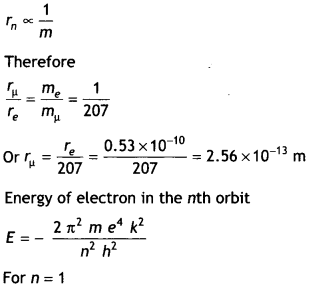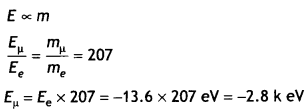Question 3.
The electron in a given Bohr orbit has a total energy of – 1.5 eV. Calculate Its
(a) kinetic energy.
(b) potential energy.
(C) the wavelength of radiation emitted, when this electron makes a transition to the ground state.
(Given Energy in the ground state = – 13.6 eV and Rydberg’s constant = 1.09 × 1o7 m-1) (CBSE Delhi 2011C)
Total energy of the electron In a Bohr’s orbit is – 1.5 eV

We know that kinetic energy of the electron in any orbt is half of the potential energy in magnitude and potential energy is negative
(a) Total energy = kinetic energy + potential energy
– 1.5 = Ek – 2Ek
1.5 = Ek

(b) Ep = – 2 × 1.5 = – 3 eV

(c) Energy released when the transition of this electron takes place from this orbit to the ground state
= – 1.5 – (- 13.6)
= 12.1 eV
= 12.1 × 1.6 × 1019 = 1.936 × 10-18 J

Let be the wavelength of the Light emitted then,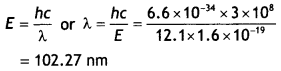Question 4.
In a Geiger—Marsden experiment, calculate the distance of closest approach to the nucleus of Z = 80, when an a-particle of 8 MeV energy Impinges on it before It comes momentarily to rest and reverses its direction. How will the distance of the closest approach be affected when the kinetic energy of the a-particle is doubled? (CBSEAI 2012)
Given Z = 80, E = 8 MeV = 8 × 106 × 1.6 × 1019 J, ro =?
Using the expression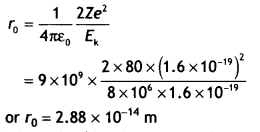When the kinetic energy of a-particle has doubled the distance of the closest approach becomes half its previous value, i.e. 1.44 × 1014 m

Question 5.
The ground state energy of the hydrogen atom is – 13.6 eV. If an electron makes a transition from an energy level – 0.85 eV to – 3.4 eV, calculate the wavelength of the spectral line emitted. To which series of hydrogen spectrum does this wavelength belong? (CBSE AI 2012)
Energy released = – 0.85 – (- 3.4) = 2.55 eV = 2.55 × 1.6 × 10-19 J
Using E = hc/λ we have
λ = $$\frac{h c}{E}=\frac{6.62 \times 10^{-34} \times 3 \times 10^{8}}{2.55 \times 1.6 \times 10^{-19}}$$ = 4.87 × 10-7 m

It belongs to the Balmer series.

Question 6.
A hydrogen atom in the third excited state de-excites to the first excited state. Obtain the expressions for the frequency of radiation emitted in this process.
Also, determine the ratio of the wavelengths of the emitted radiations when the atom de-excites from the third excited state to the second excited state and from the third excited state to the first excited state. (CBSEAI2019)
We know that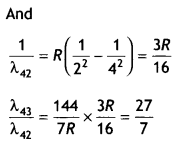Question 7.
Obtain the expression for the ratio of the de Broglie wavelengths associated with the electron orbiting in the second and third excited states of the hydrogen atom. (CBSE Delhi 2019)
We know that
2πr = nλ ….(i)
For the second excited state (n = 3)
r = 0.529(n)² A = 0.529(3)²

Putting in (i) we get 2π(0.529)(3)² = 3 λ2

For third excited state n = 4
r = 0.529 (4)²
Putting in (i) we get 2π (0.529)(4)² = 4 λ3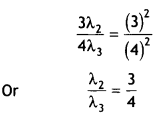Question 8.
(a) The radius of the innermost electron orbit of a hydrogen atom is 5.3 × 10-10 m. Calculate its radius in n = 3 orbit.
(b) The total energy of an electron in the first excited state of the hydrogen atom is 3.4 eV. Find out its (i) kinetic energy and (ii) potential energy in this state. (CBSE Delhi 2014C)
(a) The radius is given by
rn = $$\frac{n^{2} h^{2}}{4 \pi^{2} m e^{2} k}$$ = n²ao

where n is the number of orbit, hence r3 = 5.3 × 10-10 × 32 = 4.77 × 10-9 m

(b) Total kinetic energy = + 3.4 eV
Total potential energy = – 6.8 eV

Question 9.
Given the ground state energy E0 = – 13.6 eV and Bohr radius a0 = 0.53 Å. Find out how the de Broglie wavelength associated with the electron orbiting in the ground state would change when it jumps into the first excited state.
The de-Broglie wavelength is given by 2πrn = nλ.
In ground state, n = 1 and ro = 0.53 Å, therefore, λo = 2 × 3.14 × 0.53 = 3.33 Å

In first excited state, n = 2 and r1 = 4 × 0.53 Å = 2.12 Å, therefore,
r1 = (2 × 3.14 × 2.12)/2 = 6.66Å

Therefore, λ1 – λo = 6.66 – 3.33 = 3.33 Å.
In other words, the de-Broglie wavelength becomes double.

Question 10.
When is the Hα line in the emission spectrum of hydrogen atom obtained? Calculate the frequency of the photon emitted during this transition. (CBSE AI 2016)
Hα is obtained when ni = 3 and nf = 2Question 11.
A 12.5 eV beam is used to excite a gaseous hydrogen atom at room temperature. Determine the wavelength and the corresponding series of lines emitted. (CBSE AI 2017)
Given the energy of electron beam E = 12.5 eV
This will make the energy of the electrons 12.5 – 13.6 = – 1.1 eV.
Thus, the electrons of hydrogen will be raised to the third orbit.
Lines emitted will be ni = 3, nf = 2 Balmer series.

The wavelength is given by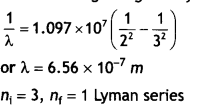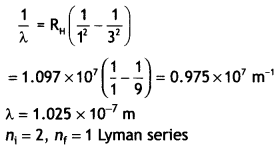For first member ni = 2, therefore, the wavelength of the first member of the Lyman series.Question 12.
Calculate the longest wavelength of the photons emitted in the Balmer series of the hydrogen spectrum. Which part of the e.m. spectrum does it belong to? [Given Rydberg constant, R = 1.1 × 107 m-1 J] (CBSE Delhi 2017C)
The longest wavelength is obtained when the electron jumps from ni = 3 to nf = 2 in the Balmer series. Therefore we have,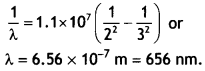It lies in the visible region.

Question 13.
10 kg satellite circles earth once every 2 h in an orbit having a radius of 8000 km. ‘ Assuming that Bohr’s angular momentum postulate applies to satellites just as it does to an electron in the hydrogen atom, find the quantum number of the orbit of the satellite. (NCERT Exemplar)
From Bohr’s postulate, we have mvn rn = nh/2π
Here m = 10 kg and rn = 8 × 106 m

We have the time period T of the circling satellite as 2 h.
That is T = 7200 s
Thus the velocity vn = 2πrn/T

The quantum number of the orbit of the satellite
N = (2πr)² × m/(T × h).

Substituting the values,
n = (2π × 8 × 106 )² × 10/(7200 × 6.64 × 10-34)
= 5.3 × 1045

Note that the quantum number for the satellite motion is extremely large. In fact, for such large quantum numbers, the results of quantization conditions tend to those of classical physics.

Question 14.
Consider two different hydrogen atoms. The electron in each atom is in an excited state. Is it possible for the electrons to have different energies but the same orbital angular momentum according to the Bohr model?
No, because according to the Bohr model, E = – 13.6 /n² eV and electrons having different energies belong to different levels having different values of n.

So, their angular momenta will be different, as nh/2π = mvr

Question 15.
Using the Bohr model, calculate the electric current created by the electron when the H-atom is in the ground state. (NCEFU Exemplar)